The tram

The tram is moving with acceleration a = 0.3m/s2. How long it will pass the first meter of track? How long does it take 10 meters. What is its speed at the end of the 10 meters track?

Result

t1 =  2.582 s
t2 =  8.165 s
v2 =  2.45 m/s

Solution:Leave us a comment of example and its solution (i.e. if it is still somewhat unclear...):Be the first to comment!Next similar examples:

1. GravitationFrom the top of the 80m high tower, the body is thrown horizontally with an initial speed of 15 m/s. At what time and at what distance from the foot of the tower does the body hit the horizontal surface of the Earth? (use g = 10 ms-2)
2. G forcesCalculate deceleration of car (as multiple of gravitational acceleration g = 9.81 m/s2) which occurs when a car in a frontal collision slows down uniformly from a speed 111 km/h to 0 km/h in 1.2 meters trajectory.
3. Free fallThe free fall body has gone 10m in the last 0.5s. Find the body speed at the moment of impact.
4. Speed of sound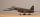The average speed of sound is 330 meters per second. Estimate how long it will hear the church bell 1 km away. Calculate the distance from what would hear sound after 10 seconds.
5. Cars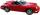Cars started at 7:00 AM. From point A car run at 40 km per hour, from B car run 70 km per hour. Between A and B is 250 km long road. When and where will meet (measured from point A)?
6. Round-tripA woman works at a law firm in city A which is about 50 miles from city B. She must go to the law library in city B to get a document. Find how long it takes her to drive​ round-trip if she averages 40 mph.
7. Cenda and Pepa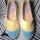Cenda and Pepa went to the event. Cenda started alone. Canda started after him for 20 minutes. How long took Cenda to catch him? Cenda traveling at 15 km/h, and Pepa traveling at 25 km/h.
8. Two boats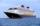The sightseeing boat sail at 25 km / h. The cruise take 6 hours. How long the another boat sails at 60 km/h?
9. Inter city busThe inter city bus leaves Suva at 10.00am and reaches Nadi at 1.00pm covering a distance of 219km. How long did it take to reach the bus Nadi?
10. Cars 6At 9:00 am two cars started from the same town and traveled at a rate of 35 miles per hour and the other car traveled at a rate of 40 miles per hour. After how many hours will the cars be 30 miles apart?
11. Two trains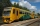From station A, the freight train traveled at a speed of 40 km/h in 9h. When he drove 15km, the fast train started from station A in the same direction at a speed of 70km/h. When will it the freight train catch up?
12. Cars 2Opava is 360 km distance from Prešov. At 8:00 hour two cars started against each other from this cities at average speeds 88 km/h and 121 km/h. At what hour its will meet and what distance its travel if car started from Prešov is faster?
13. StormSo far, a storm has traveled 35 miles in 1/2 hour in direction straight to observer. If it is currently 5:00 p. m. and the storm is 105 miles away from you, at what time will the storm reach you? Explain how you solved the problem.
14. Mail trainThe speed of mail train is 1370 meter per minute. Express it in miles per hour correct to three significant figure . Given that 1 meter =39.37 inches.
15. Bob traveled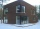Bob traveled 20 meters to Dave's house. He arrived in 3 minutes. What was his average speed for the trip.
16. Train delayThe train should be in the station for 10 minutes. It still has 32 km left. What is the expected delay if the train travels in 2 minutes 3 km except for the last section where it is 2 km in 5 minutes.
17. Average speedMichal went out of the house by car at speed 98 km/h. He came into the goal place for 270 minutes. Determine the distance between the two places.